Module 13   Integrated Financial ModellingChapter 8

# Asset Schedule (Part 2)

View chapters →

## 8.1 – Hello depreciation

We closed the previous chapter with our first balance sheet projection, i.e. the gross block. This chapter will complete the asset schedule and plug the numbers back to the P&L and Balance sheet.

At this stage, here is how the asset schedule looks  –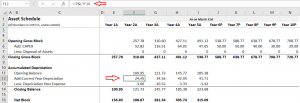The gross block looks tidy. We will now have to work our way through depreciation. The biggest challenge with forecasting the accumulated depreciation is getting the current year depreciation number, which, as you realise, flows from the P&L statement.

Here is the snapshot of current year depreciation as stated in the P&L –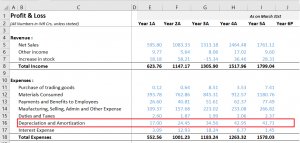Investors are often confused about these two depreciation numbers, i.e. the accumulated depreciation stated in the balance sheet and the current year depreciation number displayed in the P&L (on the expense side).

The current year depreciation stated in the P&L is the depreciation value (in Rupee terms) applicable only for the financial year under consideration. The company’s finance team calculates the current year depreciation by factoring in all the assets (gross block) on its books. The current year depreciation stated in the P&L changes for each year based on how the gross block changes.

On the other hand, the accumulated depreciation in the balance sheet is on a cumulative basis. The depreciation number gets rolled over on year on year basis. In other words, the current year depreciation (from P&L) gets added over to the next year depreciation, thus forming the accumulated depreciation for this year.

Don’t worry if you find this confusing; you will understand this better shortly, but for now, I want to you think about the direction we are heading in.

We have already projected the Gross block number. If we can project the current year depreciation number in the P&L, we can apply the base rule again in the asset schedule and forecast the accumulated depreciation number.

After we forecast the accumulated depreciation, we can also calculate the netblock of the company. Finally, the net block number from the asset schedule flows back to the balance sheet.

By carrying out the above steps, we achieve two things –

• Project the current year depreciation number in P&L
• Get the projected netblock number in the asset schedule, which gets plugged back to the balance sheet

Everything depends on techniques to forecast the current year depreciation.

Before we proceed, a slight but relevant digression 😊

I want you to think about the following situation. Assume you are a freelance photographer with a variable monthly income. Your income depends on the work that comes your way.  You are also responsible for managing your household expenses.Your monthly income for October is 25K, of which you spend 3K on entertainment.

The next month you earn 30K. How much do you think you should spend on entertainment for the month of November?

The easiest way to do this is to spend in the same proportion as you spent in October.

In October –

3000/25000

= 12%

Hence for September,

12% * 30000

= 3600

We have used the method of proportions here. I want to extend this thought and project depreciation for the current year.

For Year 5, Depreciation and Amortization stated in P&L is 41.71Cr. The gross block, as stated in the balance sheet for Year 5, is 538.76Cr. The projected Gross Block for Year 6 is 588.77 Crs. Given this, what do you think will be depreciation for Year 6?

Let us apply the proportion technique.

For gross block worth 538.76Cr, the company reported depreciation of 41.71Cr, which means –

41.71/538.76

= 7.74%

For Year 6, the gross block value is 588.77 Crs, so what is the depreciation given the same proportion?

7.74% * 588.77

= 45.58Cr

With this, we can estimate that the depreciation for the next year would be 45.58Crs. Remember, this number flows into the P&L.

The depreciation value will remain the same at 7.74%.

You can extend this a bit more. Instead of taking the previous year’s proportion and assuming the same proportion will hold for the next year, you can calculate the depreciation to gross block ratio for all the historical years and then take the five years rolling average for the future years.

It will look something like this –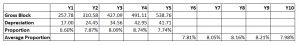Y6 = Average of Y1 to Y5

Y7 = Average of Y2 to Y6

Y8 = Average of Y3 to Y7

So on and so forth.

You can choose either technique; I’ll stick to the first technique for simplicity.

Of course, the 3rd alternative is to dig deep into gross block and get into the accountant shoe to figure the exact depreciation value; you can do that if you have a strong accounting background.

Going back to the P&L with the 1st technique, I can directly input the formula –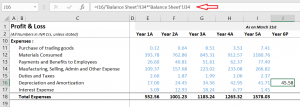As you can see, in cell J16, which points to the Depreciation expense for Year 6, I’ve divided the depreciation amount stated in P&L for year five over the gross block stated in the balance sheet for year 5. The result of this division is the depreciation proportion, which I then multiply by the gross block projected for Year 6.

The resulting value is the depreciation amount for Year 6. Note, this is a projection that we are making. I can extend the same math to all the future years and get the depreciation expense for the year.

By the way, we also made our first P&L projection, so it’s a tiny baby step in our financial modelling journey 😊

At this point, my P&L looks like this –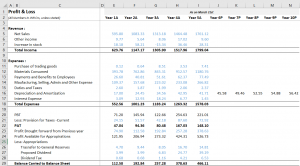Alright, with this in place, let’s go back to the asset schedule.

## 8.2 – Accumulated depreciation

Some of you may have guessed the next few steps already.  It is pretty simple, we go back to the asset schedule sheet and plug in the current year depreciation number from P&l. Once we have that, we use the base rule to complete the accumulated depreciation.

Here is how I’ve plugged the number from P&L –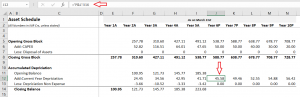We have the closing balance for Y5, i.e. 223.68Cr, which becomes the opening balance for Y6. Add to this the current year depreciation, and we should get the closing balance for Y6. Of course, we won’t get into the depreciation non-expense bit as there is no visibility on this line item. Hence will keep it at zero for all the future years.

I’ve applied the base rule to get the closing balance of accumulated depreciation. The netblock is the gross block – accumulated depreciation, which I’ve projected for future years.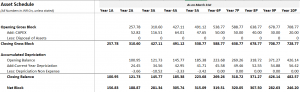Of course, you take the accumulated depreciation number from the asset schedule and complete the netblock calculation in the balance sheet.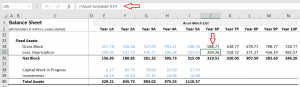With this, we have projected the Fixed assets section in the balance sheet.

In the next chapter, we will discuss the debt schedule.

### Key takeaways from this chapter

• Accumulated depreciation stated in the balance sheet is on a cumulative basis.
• The current year depreciation stated in the P&L is for the year only
• You can use the method of proportions to forecast the depreciation for the year
• You can apply the base rule to forecast the accumulated depreciation
• Netblock = Gross block – accumulated depreciation

1.Tanmay says:

Hello sir, do you know the fact, Zerodha don’t need to invest their money on marketing, because of the trust that Nikhil Sir, Nitin kamath Sir,Karthik Rangappa Sir and all his team created among all the Janta.
( this I can say after listening both brothers on TRS Podcast).

Seriously thanks a lot sir for all this Hardwork that you all are doing consistently. I have started investing a year ago because of this varsity, now i have started Financial Modelling because of this only.
Blessings From My side Sir!!
And one more thing please , provide us some virtual projects or interns ( If possible, No paid chalega XD) so that we can learn more,because at the end what actually matters is ” Kaam Aana Chaiye”

•Karthik Rangappa says:

Thanks for the very kind words, Tanmay. I’m glad you liked the content on Varsity 🙂 About projects, I’ve been trying to nudge ppl to take up tasks in the Financial modelling module. Hopefully, that will help 🙂

2.AZEEM says:

What is the significance of net block?

•Karthik Rangappa says:

Net block = Gross block – Accumulated depreciation.

Basically gives you a sense of how the compnay’s fixed assets are growing.

3.Harsh Dhiman says:

Because of this module principles , i have gained the conviction to stay invested in a company i chose..
Thanks sir

•Karthik Rangappa says:

Happy learning, Harsh!

4.Kamlesh says:

Hello Karthik,
So, I was going through the Asset Schedule of Apollo and there the data is in PP&E instead of Gross Block and also, there is no such Depriciation item in Balance sheet so for that reason what I did is assumed the PP&E in B/s as Net block and used it to reverse it for Gross block using Depriciation given in Income statement.
So this is how it looked:

Opening PP&E :
Less : Depriciation : (Took the Current depriciation amount)
Add – Acquisitions : (Given in Operating cash flow)
Any Changes :
Closing PP&E : (Assumed as Net block)

(But there is a difference in the amount in asset schedule amount and balance sheet amount, so should I write it off balancing the amount and writing it off with “Any changes”)

Sorry for a such a lenghty query but was applying your model method for a competition.
Thankyou again.

•Karthik Rangappa says:

Kamlesh, so if you are considering PP&E as netblock, then why are you deducting depreciation again? Net block is what you get after deducting depreciation right?

5.Shivansh Agarwal says:

the company which I have taken has not given the accumulated depreciation in its balance sheet, the only place where deprecation is mentioned in its financial statements is in p&l statement under expenses

and yea! one more thing the company which I have chosen, in their balance sheet they have not clubbed assets under gross block (there is no such thing like gross block and net block in the balance sheet) they have given each asset and its value separately in the balance sheet so for the gross block what I did was I went deep into the statements and found out the gross value

so what should I do for the accumulated depreciation

•Karthik Rangappa says:

Shivansh, please check the associated notes. They should have mentioned the accumulated depreciation in the balance sheet. Even the gross block.

6.Raghavendra Prabhu says:

Sir give the model of complete excell sheet

•Karthik Rangappa says:

Will put up the next chapter soon.

7.Jhanvi says:

Hello Sir,
Hope you’re doing well. Firstly I’d like to thank you from the very depth of my heart for these modules. I’ve learnt alottttt and it shows the level of hard work and dedication you put in your work.
Just wanted to know when will you be adding further chapters to this module.
(Waiting eagerly :))

•Karthik Rangappa says:

Thanks for the kind words, Jhanvi. I’ve been slightly busy over the lat few days. Will put up the next chapter in the coming week.

8.Shivansh Agarwal says:

in the balance sheet of my company they have not given the net block or gross block (instead they have written each asset separately) but they provided gross block and net block information related to the assets in the notes, related to the balance sheet in the annual report. So if I want to add the net block or gross block in the balance sheet how should I do it cause we have calculated the values for the entire balance sheet not for separate assets

•Karthik Rangappa says:

Shivansh, you will have to rebuild the assets bit by taking the gross block and depreciation values from the notes. Btw, why are you adding net block to gross block? Gross block – depreciation = Net block.

9.Shivansh Agarwal says:

no no no…… I am not adding net block to gross block I am not that bad student 😂😂 of such a good teacher, I wrote something wrong which I didn’t meant

and for the query can I make my separate balance sheet where l will replace the gross block assets in the balance sheet with gross block

•Karthik Rangappa says:

Got it, yes, you can expand the balance sheet and include the gross block. You can do it as long as it does not affect the over all balance of the balance sheet 🙂

10.Shivansh Agarwal says:

while creating a separate balance for gross block for which we had a chat earlier, while doing the same I found that in the balance sheet for FY2021 the other intangible asset is given as (net carrying amount= 3455 million) BUT in the notes related to it the net carrying amount is calculated as (3456 million) so which amount should I take

•Karthik Rangappa says:

You should consider the number as stated in the Balance sheet. By the way, it’s a very small difference, so not to worry.

11.Anurag says:

Hello Sir, could you please also cover how to go about Intangible Asset Schedule projection? Would it be in a similar manner?

•Karthik Rangappa says:

It would be. But come to think of it, I’ve never projected intangible assets. Need to spend some time thinking through this.

12.Nitish Bhushan says:

Hello Karthik. The source for CAPEX is Reserves & Surplus – is this correct?

•Karthik Rangappa says:

Not necessarily. The company can choose to borrow, do an IPO, do an FPO, or even a rights issue.

13.Sonal says:

Hi Sir,

I am working on the Manufacturing company of “CEAT tyres”, there is no depreciation in the balance sheet, the depreciation is given only in P&L statement.
How should I calculate accumulated depreciations?
The current year depreciation I have taken from the P&L statement , but opening balance depreciation from where should I consider, as it is directly not available in the balance sheet. However, I have checked the associated notes in the annual reports, but still not getting the clarity.

•Karthik Rangappa says:

It is not possible. If there is depreciation in P&L, there must be a corresponding entry in the balance sheet. You need to check the notes associated with the gross block or net block to get the depreciation value.

14.Sonal says:

Hi Sir,

There is no entry for depreciation in balance sheet, below are the items mentioned in the balance sheet of “CEAT Tyres”

Non-current assets
Fixed assets
Tangible assets
Intangible assets
Capital work-in-progress
Intangible Assets Under Development
Total fixed assets

However, I have checked the associated notes, the notes has been divided in three columns i.e Cost , Depreciation and net value, they have shown the depreciation of each item , but I am not getting the exact calculation, that which amount to be consider for calculating.

Can you please help me, how should I arrived with the exact amount of depreciation from the associated notes, so that I can deduct the depreciation amount from the gross block to get the net block.

•Karthik Rangappa says:

So from the associated notes, you will get the opening value of the depreciation (Which is the closing value of the previous year), the current year’s depreciation (as stated in the P&L), and the closing value; this will be made available for both tangible and intangible assets. The format is the same as the base rule we have learned in this module. So from the given split up, you should be able to net the total depreciation for the year.

15.Sonal says:

Hi Sir,

By looking at the associated notes, I am not able to identify exact depreciation, from the associated notes , it’s bit confusing, can you please help me to sort this, if possible.

•Karthik Rangappa says:

Sonal, look for the note number of the Net block. Goto net block notes and see how they are calculating it. They would start with the gross block, deduct the depreciation, and then calculate the netblock (maybe for both tangible and intangible assets). From this you can build the gross block schedule.

16.paras says:

Few questions:-

1)When we talk about CapEx, in the annual reports the data is been provided of each asset on individual basis and just as we did the calculation to derive the CapEx the same is already been provided for each asset.So,do we have to consider each asset while preparing the asset schedule or we need to consider the total values in whole,i.e.inclusive of all assets?

2)If we tend to consider each asset the how can we forecast based on Management’s discussion as in the case of Bajaj Auto Ltd,the CFO had given a rough amount of Rs.250-300 Crs as a whole?

3)If we were to forecast the CapEx for each individual asset,could it be based on historical trend?

4)In case of calculation of depreciation for P&L can’t we do the reverse calculation,i.e taking accumulated depreciation closing balance as a % of gross block and then subtracting closing values from opening values.
PS: I tried it, seem’s like not a good idea though as some value may also come in negative.

Do guide for the same.

•Karthik Rangappa says:

1) You can consider CAPEX as a whole. But if the data is consistent and clean, you can consider individual line items also. But this is a lot more work 🙂
2) That won’t be possible. Taking each line item is not feasible for most of the companies
3) Yes, based on historical trends and projections basis that

15 chapters

22 chapters

16 chapters

13 chapters

25 chapters

14 chapters

7 chapters

19 chapters

16 chapters

16 chapters

32 chapters

603 chapters

18 chapters

9 chapters